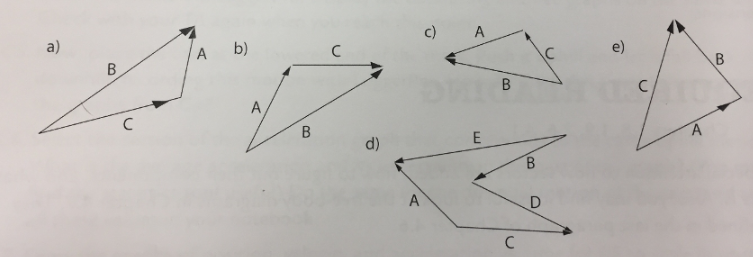# Problem: Write a vector equation for A for each arrangement of vectors. For example, if adding B and C gives A then write A=B+C. Each answer you give should start with A =.

###### FREE Expert Solution

Moving from tail to head gives a positive vector while moving from head to tail gives a negative vector.

a)

A = - C + B = B - C

b)

A = B - C

95% (349 ratings)###### Problem Details

Write a vector equation for A for each arrangement of vectors. For example, if adding B and C gives A then write A=B+C. Each answer you give should start with A =.